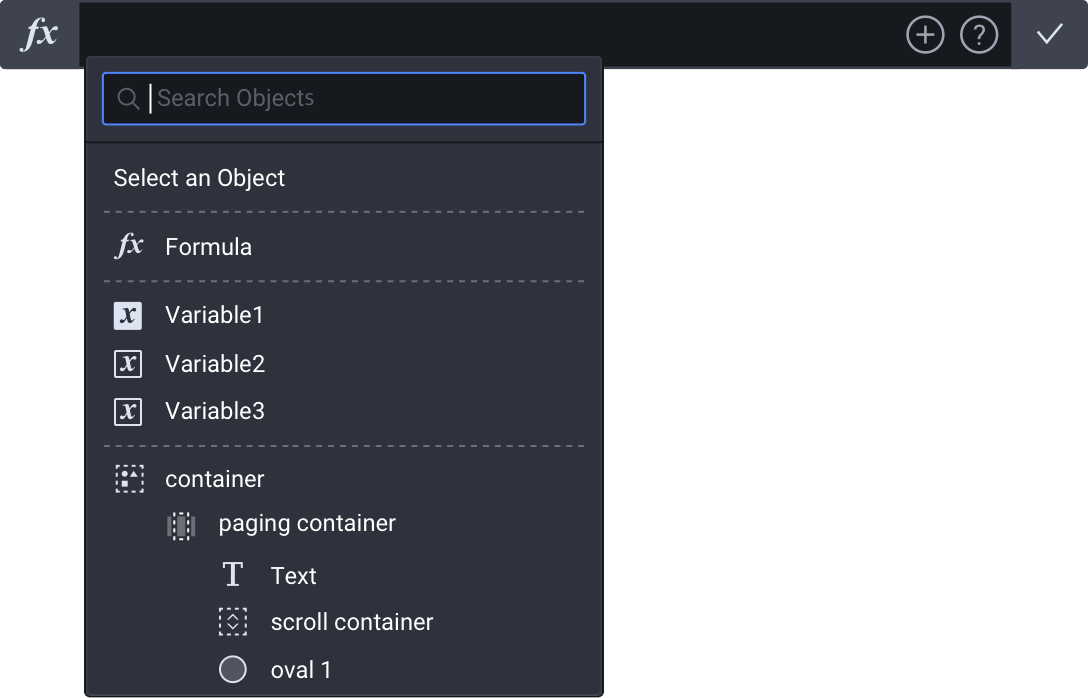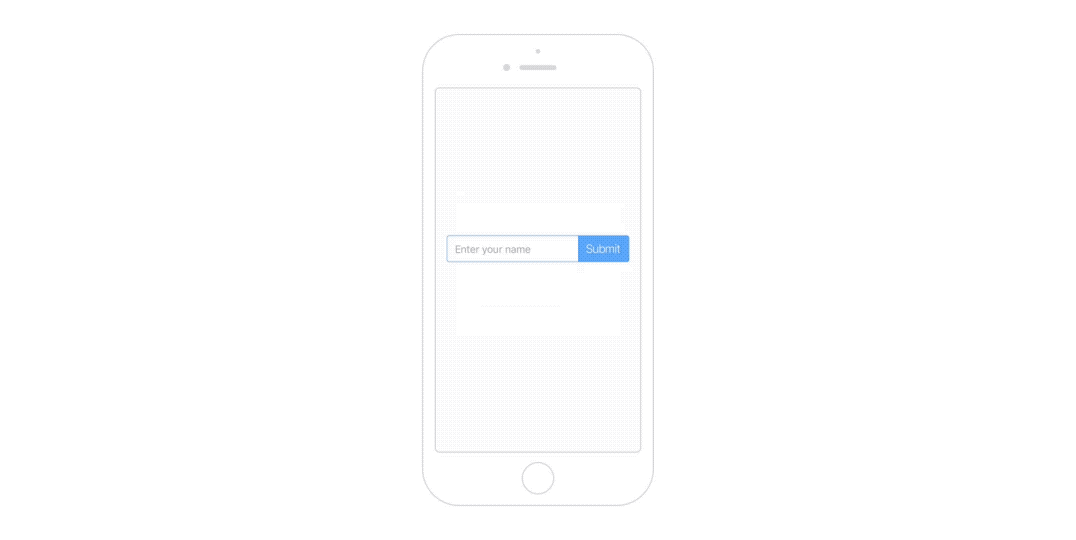# Formulas

Formulas and variables make it possible for designers to make prototypes with interactions that are more dynamic. You are able to define your own variables and create your own formulas where you can use arithmetic operations, variables and functions, and even access layer properties. These all unlock the potential of interactions that are far more dynamic than what you are used to.

### Formula Input

The formula input field is where you can create formulas. You can include layer properties, variables and functions simultaneously in the formula input field. Clicking on the plus icon will help you select the variables and layer properties to autocomplete formulas.## Basic Tutorials

### Formula

The basics of formulas are explained in this video showing how useful they can be. For example, you can use coordinates of a layer directly in an interaction making it dynamic, instead of using fixed values.

### Variable

The basics of variables are explained in this video showing how easy it is to use them. You have various types of variables that can hold different kinds of values. Subsequently, you can use variables in various ways.

## Examples

Besides fixed values, it is possible to use formulas for responses. Layer properties can be utilized when it comes to formulas. The x-coordinate of each "menu" layer is utilized in the formulas for the Move responses, in order to achieve an interaction where the blue layer moves left and right, as seen below.### Spinner

You can use variables to create a simple spinner that increases by one or decreases by one.### Ripple Effect

Coordinates of a point where a touch is registered can be used through predefined variables. In order to create a ripple effect at the exact position where a touch is registered, "\$touchX" and "\$touchY" are used in the Move response of the ripple layer.### Text Input

The text property of the input layer refers to the text that was entered through the input layer. With functions, you are able to combine texts and manipulate texts to your liking.